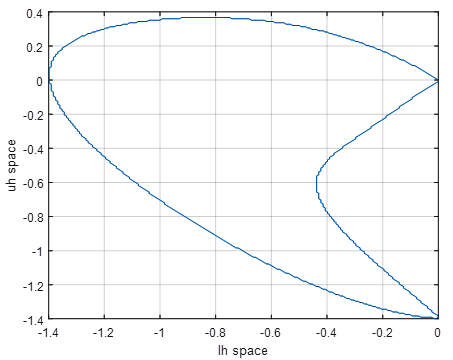# A New Multi-Step Method for Solving Delay Differential Equations using Lagrange Interpolation

## Authors

• V. J. Shaalini Department of Mathematics, Bishop Heber College, Trichy, India
• S. E. Fadugba Department of Mathematics, Ekiti State University, Ado Ekiti, Nigeria

## Keywords:

Multi-step method, delay differential equations, interpolating function, Lagrange interpolation, stability polynomial and stability region.

## Abstract

This paper presents 2-step p-th order (p = 2,3,4) multi-step methods that are based on the combination of both polynomial and exponential functions for the solution of Delay Differential Equations (DDEs). Furthermore, the delay argument is approximated using the Lagrange interpolation. The local truncation errors and stability polynomials for each order are derived. The Local Grid Search Algorithm (LGSA) is used to determine the stability regions of the method. Moreover, applicability and suitability of the method have been demonstrated by some numerical examples of DDEs with constant delay, time dependent and state dependent delays. The numerical results are compared with the theoretical solution as well as the existing Rational Multi-step Method2 (RMM2).

Dimensions

Y. Kuang, Delay differential equations with applications in population dynamics, Academic Press, Boston, San Diego, New York. (1993).

E. Fridman, L. Fridman & E. Shusti, “Steady modes in relay control systems with time delay and periodic disturbances”, Journal of Dynamical Systems Measurement and Control 122 (2000) 732.

I. Epstein & Y. Luo, “Differential delay equations in chemical kinetics: non-linear models; the cross-shaped phase diagram and the oregonator”, Journal of Chemical Physic 95 (1991) 244.

R.K.Saeed&B.M.Rahman,“Adomiandecomposition method for solving system of delay differential equation”, Australian Journal of Basic and Applied Sciences 4 (2010) 3613.

H. M.Radzi, Z. A. Majid, F. Ismail & M. Suleiman, “Two and three point one-step block method for solving delay differential equations”, Journal of Quality Measurement and Analysis 8 (2012) 29.

F. Ishak, M. B. Suleiman & Zanariah A. Majid, “Block Method for solving Pantograph-type functional differential equations”, Proceeding of the World Congress on Engineering 2 (2013).

T. A. Biala, O. O. Asim & Y. O. Afolabi, “A combination of the Laplace and the variational iteration method for the analytical treatment of delay differential equations”, International Journal of Differential Equations and Applications 13 (2014) 164.

A. E. K. Puhpam & J. V. Shaalini, “Solving delay differential equations with constant lags using RKHaM method”, International Journal of Scientific Research 5 (2016) 585.

G. A. Bocharov, G. I. Marchuk & A .A. Romanyukha, “Numerical solution by LMMs of stiff delay differential systems modelling an immune response”, Numerische Mathematik 73 (1996) 131.

J. Vinci Shaalini & A. Emimal Kanaga Pushpam, “Analysis of Composite RungeKutta MethodsandNewOne-stepTechniqueforstiff Delay Differential Equations”, IAENG International Journal of Applied Mathematics 4 (2019) 359.

J. V. Shaalini & A. E. K. Pushpam, “A new one step method for solving stiff and non-stiff delay differential equations using Lagrange interpolation”, Journal of Applied Science and Computations 6 (2019) 949.

S. O. Fatunla, “Nonlinear multistep methods for initial value problems”, Computers and Mathematics with Applications 8 (1982) 231.

K. O.Okosun&R.A.Ademiluyi, “Atwo-step second order inverse polynomial methods for integration of differential equations with singularities”, Research Journal of Applied Sciences 2 (2007) 13.

O. E. Abolarin & S. W. Akingbade, “Derivation and application of fourth stage inverse polynomial scheme to initial value problems”, IAENG International Journal of Applied Mathematics 47 (2017) 459.

T. Y. Ying & N. Yaacob, “A new class of rational multi-step methods for solving initial value problems”, Malaysian Journal of Mathematical Sciences, 7 (2013) 31.

J. Vinci Shaalini, A. & E. K. Pushpam, “A Class of 2-Step fourth order rational multi-step methods for solving delay differential equations”, International Journal for Research in Engineering Application & Management 5 (2019) 164.

S. E. Fadugba, S.N. Ogunyebi & B.O. Falodun, “An examination of a second order numerical method for solving initial value problems”, Journal of the Nigerian Society of Physical Sciences 2 (2020) 120.2021-08-29

## How to Cite

A New Multi-Step Method for Solving Delay Differential Equations using Lagrange Interpolation. (2021). Journal of the Nigerian Society of Physical Sciences, 3(3), 159-164. https://doi.org/10.46481/jnsps.2021.247

## Section

Original Research

## How to Cite

A New Multi-Step Method for Solving Delay Differential Equations using Lagrange Interpolation. (2021). Journal of the Nigerian Society of Physical Sciences, 3(3), 159-164. https://doi.org/10.46481/jnsps.2021.247